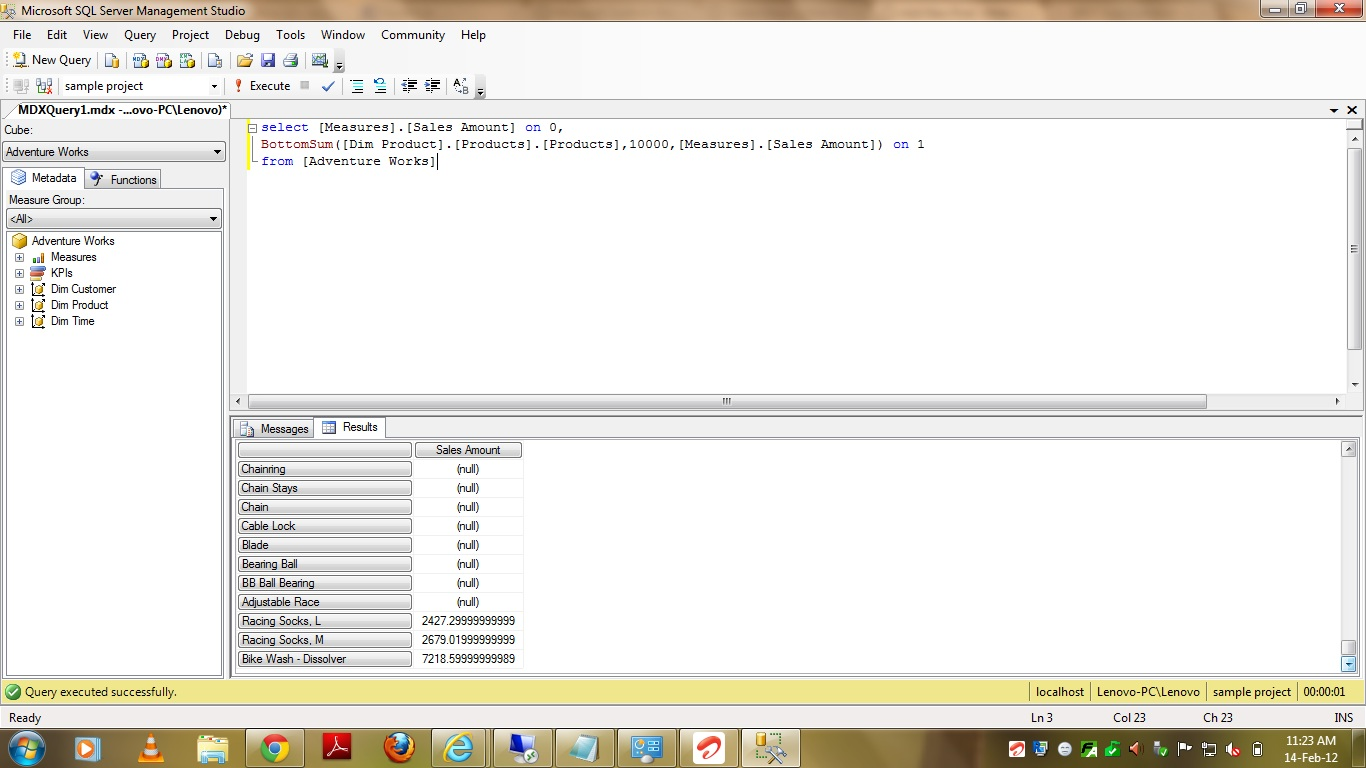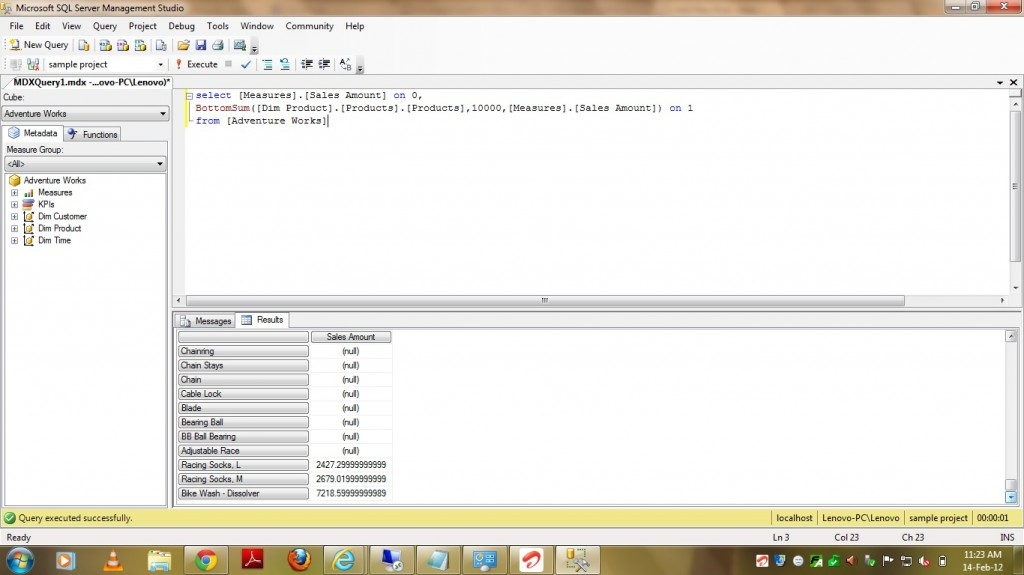# BottomSum Function in MDX

5333Friends,

This funcmtion is very much similary to the functions BottomCount and BottomPercent. Please find the Major Difference between the all three functions –

1) BottomCount – Orders the set and then returns specified number of tuples in the specified set with the lowest values will be pulled.

2) BottomPercent – Orders the set and then returns tuples from the bottom of the set with the lowest values whose cumulative total is less than or equal to the supplied percentage.

3) BottomSum – Orders the set and then returns the summed value of a numeric expression across a supplied set.

In this post we are gonna discuss about BottomSum Function. This function will do TWO jobs –

1) Sorts the set based on numeric parameter in Ascending order.

2)  Returns tuples from the bottom of the set with the lowest values whose cumulative total is less than or equal to the supplied Number.

Syntax –

BottomSum(Set_Exp,Value,Numeric_Exp)

Set_Exp – Valid MDX Set Expression

Value – It specifies the Total Value of Cumulative sum of records to be returned.

Numeric_Exp – It specifies on what column basis the set needs to be ordered.

The BottomSum function sorts a set in ascending order, then returns tuples from the bottom of the set with the lowest values whose cumulative total is less than or equal to the supplied value. This function returns the smallest subset of a set whose cumulative total is at least equal or near to the specified value. The returned elements are ordered largest to smallest.

PFB sample query Which pulls 10 Percent of Products based on Sales Amount.

select [Measures].[Sales Amount] on 0,
BottomSum([Dim Product].[Products].[Products],10000,[Measures].[Sales Amount]) on 1If you see the above screenshot, the sum of returned values looks like more than the value specified and it is becausxe that the BOTTOMSUM function returns NEAREST value to the specified value in parameters. It is returning soo many records among which most are having NULL as Sales Amount. As we are asking for Bottom Cumulative sum of records based on Sales Amount, it is pulling all the  NULL records and PFB the MDX query to eliminate NULLs from output –

select [Measures].[Sales Amount] on 0,
BottomSum(Filter([Dim Product].[Products].[Products],
NOT ISEMPTY([Measures].[Sales Amount])),10000,[Measures].[Sales Amount]) on 1
This is a very useful function in cases where the user wish to see the Bottom Products (or simply least sales products) which is contributing some %ge of sales. So, Please don’t ignore it ..